# PREDDIMER

Prediction tool for an ensemble of transmembrane α-helical dimer conformations

# PREDDIMER manual

## Prediction algorithm

Starting form sequences of transmembrane (TM) α-helices forming a probable dimer (one sequence in a case homodimer and two different sequences for heterodimers), PREDDIMER algorithm performs scanning of possible superpositions of helical surfaces (or dimer conformations), estimation of their complementarity, reconstruction of a dimer structure, geometry optimization, ranking and filtering of the results (Figure 1).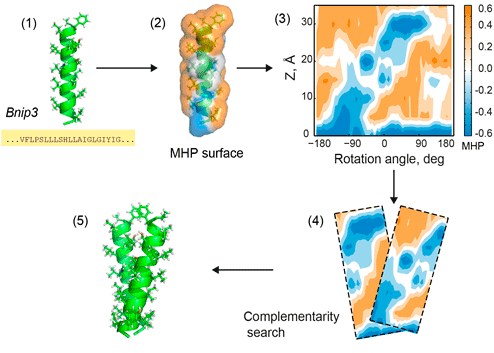Figure 1. PREDDIMER key steps. 1 — reconstruction of TM-helices from sequences; 2 — mapping of hydrophobic/hydrophilic properties on helical surfaces according to the molecular hydrophobicity potential (MHP) approach; 3 — projection of surface properties (MHP and landscape) onto the cylinder and building 2D-maps (positive values of MHP correspond to hydrophobic regions); 4 — pairwise comparisons of surface fragments using a fixed widow sliding along 2D-maps of respective helices, estimation of surface complementarity; 5 — reconstruction of 3D conformation of the dimer. All steps are shown for the TM-fragment of Bnip3 protein .

### Reconstruction of TM dimer

For each sequence, its respective TM-α-helix is constructed in an ideal conformation using the standard statistics of sidechain dihedrals. Helical surface with mapped hydrophobic/hydrophilic properties is calculated using PLATINUM software . Hydrophobicity at each molecular surface point was estimated using the molecular hydrophobicity potential (MHP) approach . Upon prediction of the dimer structure, both rotation angles (α1, α2; see Figure 2) around axes of the helices (from 0 to 360°, with 6° step) along with a number of crossing angles (χ) between the axes of the monomers (from −60 to 60, with 5° step) are systematically varied, thus giving in total about 105 variants (61×61×25).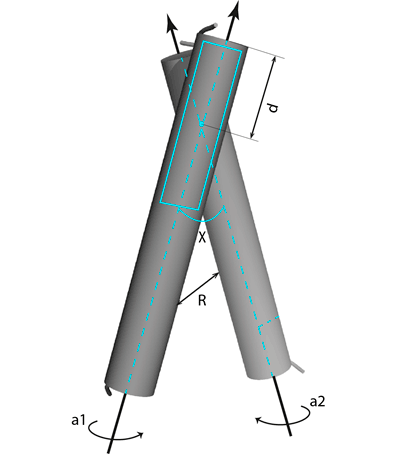Figure 2. Dimer parameters. R is distance between the helical axes; χ —crossing angle between them; d — position of a center of a sliding window (20 Å, shown with the cyan rectangle) along the helical axes; α1, α2 — rotation angles around axes of the helices.

Matching between the helical landscapes and spatial distributions of hydrophobic properties for each combination of surface patches interpolated on a regular grid and representing helical surface with respect to rotation and tilt angles were calculated using a scoring function FINT (α1, α2, χ, R, d), where R is the distance between helical axes and is the shift along the helical axis. FINT calculated as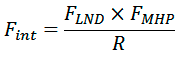where FLND and FMHP account for complementarity of landscapes and hydrophobic properties of surface patches, respectively. FMHP represents a fraction of surface points of both patches for which hydrophobicity is matched. FLND represents a fraction of points satisfying the following inequality: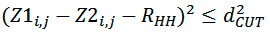where Z1i,j, Z2i,j — distances of points of both segments from helical axes (Figure 3), dCUT=1 Å and R’HH — distance between surface patches equivalent to distance between helical axes. This distance is varying by the algorithm in order to get the maximum value of FLND.Figure 3. Determination of landscape complementarity for surface patches 1 and 2.

NB. Maximum of FINT not necessarily corresponds to the best dimer conformation since at this stage the algorithm works with ideal geometry of sidechains. Final assessment of packing quality for dimer conformations is provided by the scoring function (FSCOR, see below), which is calculated for reconstructed and minimized spatial structures of a dimer.

For each angle χ, spatial structure of a dimer with the best value of FINT (α1, α2, R, d) is reconstructed (Figure 2) and geometrically optimized using a simple energy minimization procedure in vacuum (openBabel  is used for management of molecular structures).

### Calculation of dimer packing quality

For each predicted dimer conformation, packing quality of helices is evaluated using the scoring function FSCOR:where Pack is the term corresponding to a relative number of atoms packed within the structures, Int is the term accounting complementarity of hydrophobic properties on the helix—helix interface, Env is the term estimating correspondence of polar and structural properties of the dimer surface exposed to lipid environment.

Packing of atoms on the dimer interface is estimated as:where NCP is the number of backbone atom pairs, whose interhelical distance Rij is smaller than cutoff radius Rmax (8 Å).

Hydrophobic complementarity between helices is evaluated as:where NCH is the number of atom pairs, whose interhelical distance Rij is smaller than cutoff radius Rh (5.5 Å), pij is the ”hydrophobic score” depending on hydrophobicity of atom and atom j (1.0 — for contacts of the same type; −1.0 — for hydrophobic (phob) / hydrophilic (phil) contacts; 0.5 — for neutral (neut) / hydrophobic and neutral / hydrophilic contacts). Phob, phil, and neut types are defined according to the molecular hydrophobicity potential (MHP) atomic constants: MHP>0.1, MHP<−0.1, and 0.1 ≤ MHP ≥ −0.1, respectively.

Hydrophobic properties of the dimer surface are also estimated using MHP formalism: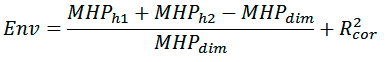where MHPh1, MHPh2, and MHPdim are the sums of MHP values on monomer and dimer surfaces, respectively; R2cor is the correlation coefficient calculated between distribution of MHP properties on the dimer surface and average distribution of MHP properties of transmembrane fragments from a model set of membrane proteins (30 non-homologous molecules). These parameters are calculated using PLATINUM software .

### Filtering of the predicted conformations

All predicted dimer conformations are clustered using pairwise calculations of backbone root-mean-square deviations (RMSD) and correlations between interhelical contact maps (Figure 4). Structures are considered similar, if they have RMSD for backbone atoms <2.5 Å and Person correlation between contact maps >0.75. Resulting filtered structures are subsequently sorted according to FSCOR values of representative models.

### Building 2D maps of interacting helices

2D MHP-maps of helices in the dimer are built as a breakdown of a cylinder with mapped MHP values that are projected from molecular surface of α-helix that is coaxial with the cylinder (see Figure 1). Individual map is built for each helix, and the contact area is shown with a contour.Figure 4. Comparison of dimer conformations using spatial coordinates (left panel) and interhelical contact maps (right panel).

1. Polyansky A.A., Volynsky P.E., Efremov R.G. (2012). Multistate organization of transmembrane helical protein dimers governed by the host membrane. J. Am. Chem. Soc. 134, 14390;
2. Pyrkov T.V., Chugunov A.O., Krylov N.A., Nolde, D.E., Efremov R.G. (2009). PLATINUM: a web tool for analysis of hydrophobic/hydrophilic organization of biomolecular complexes. Bioinformatics 25, 1201;
3. Efremov R.G., Chugunov A.O., Pyrkov T.V., Priestle J.P., Arseniev A.S., Jacoby E. (2007). Molecular Lipophilicity in Protein Modeling and Drug Design. Curr. Med. Chem. 14, 393;
4. O'Boyle N.M., Banck M., James C.A., Morley C., Vandermeersch T., Hutchison G.R. (2011). Open Babel: An open chemical toolbox. J. Cheminf. 3, 33.